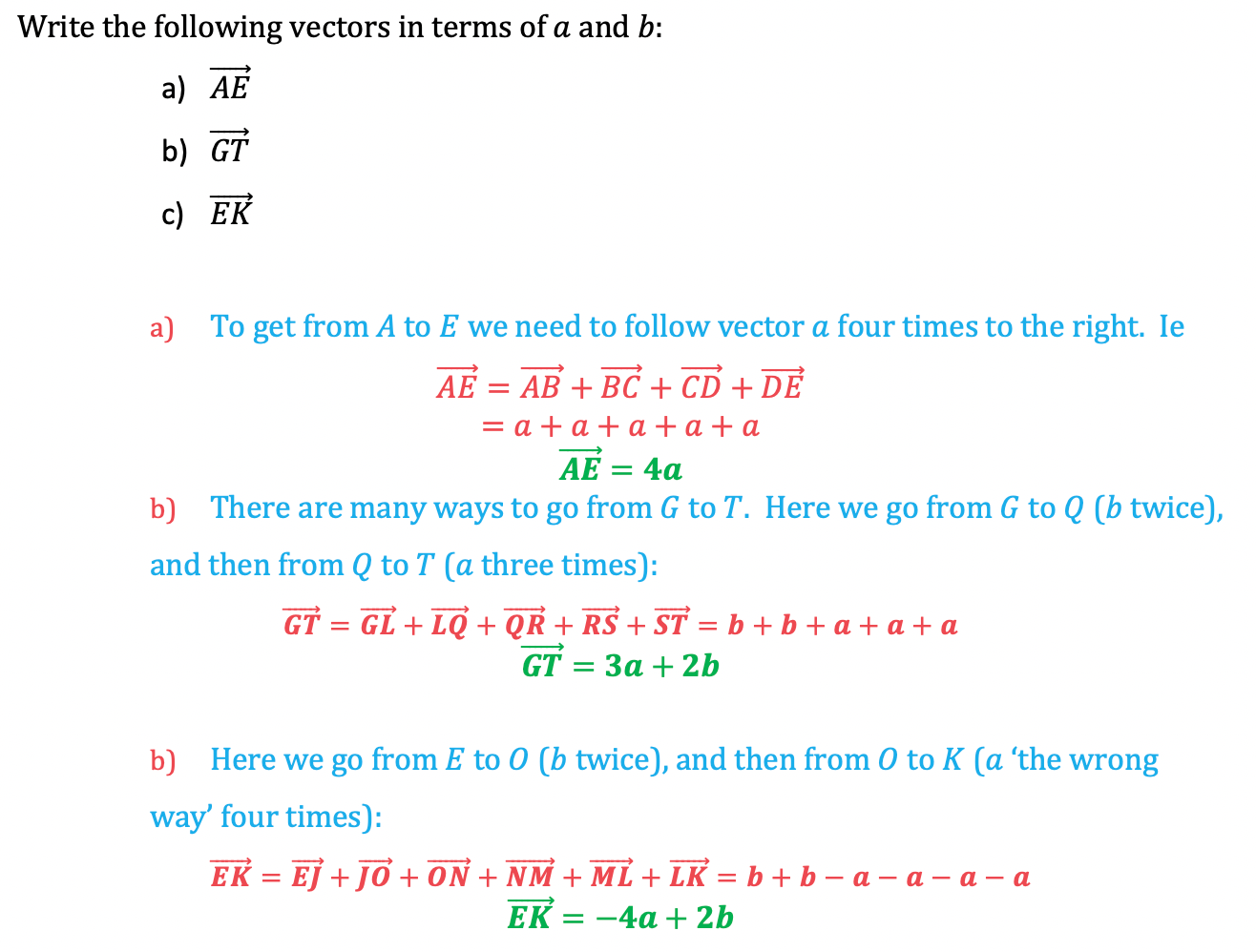# Edexcel IGCSE Maths 复习笔记 5.1.2 Vectors - Finding Paths

Edexcel IGCSE Maths 复习笔记 5.1.2 Vectors - Finding Paths

#### Finding paths in vector diagrams

• It is important to be able to describe vectors by following paths through a geometric diagram
• The following grid is made up entirely of parallelograms, with the vectors  and  defined as marked in the diagram: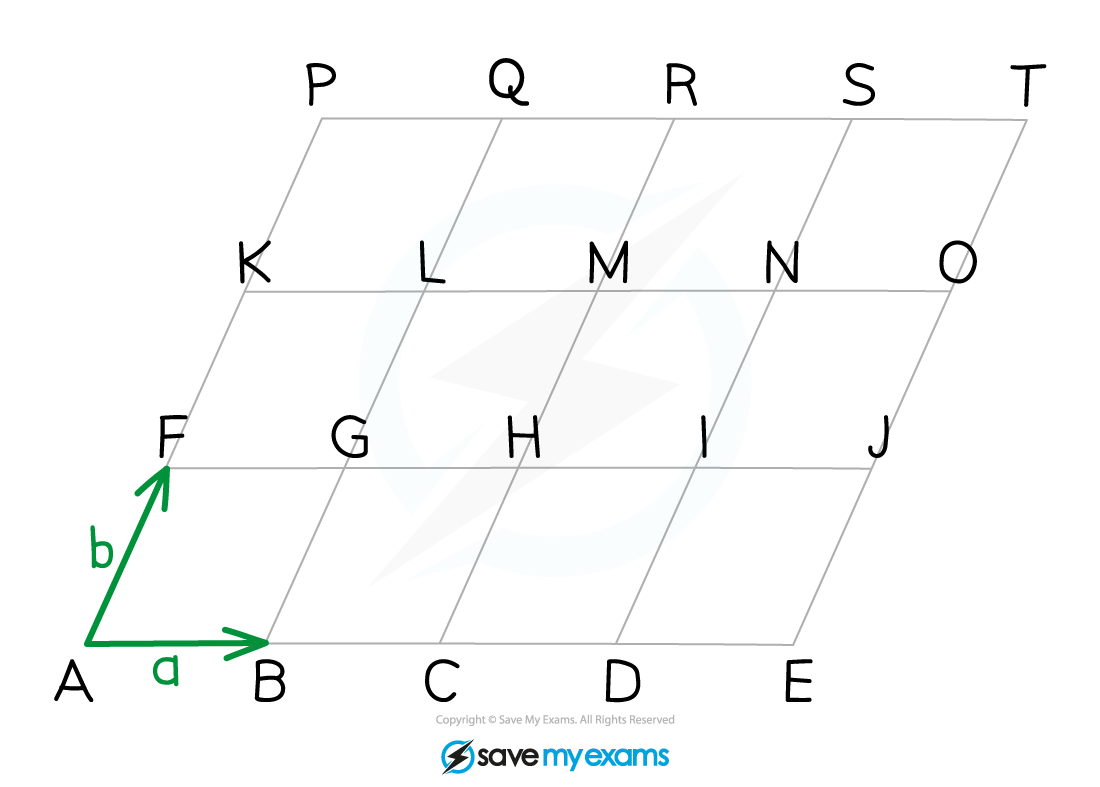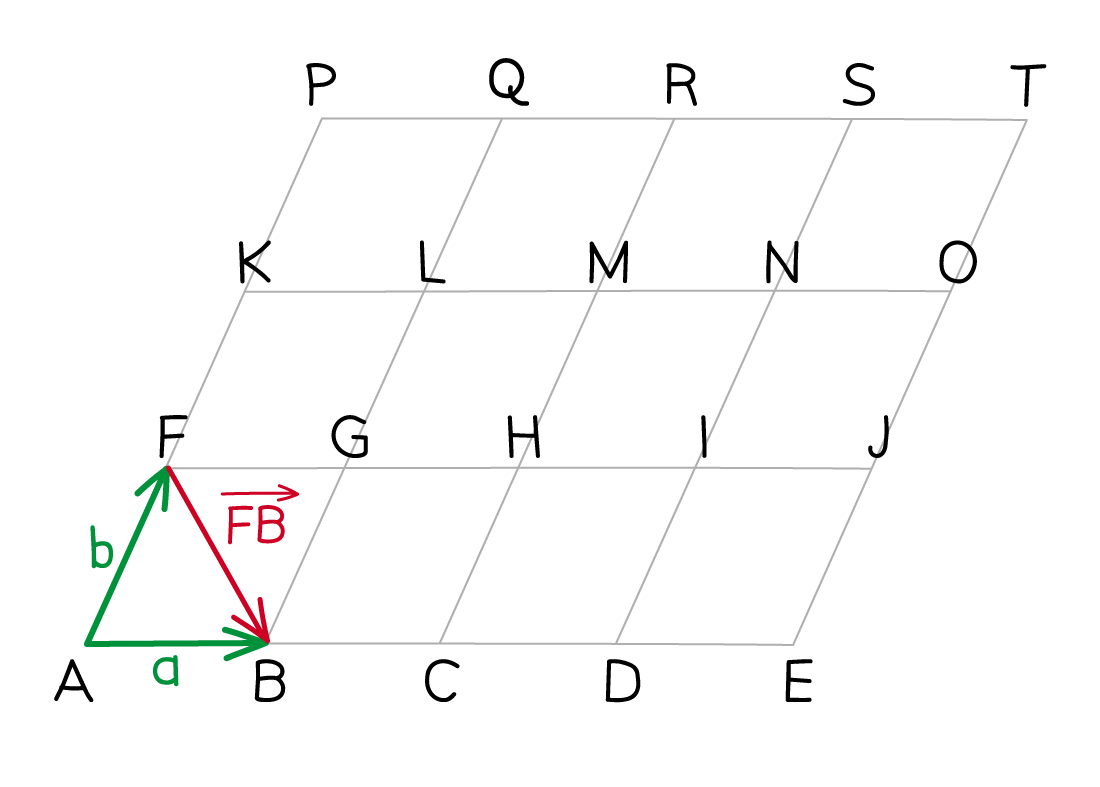• Getting from point to point  we have to go the 'wrong way' down  and then the 'right way' along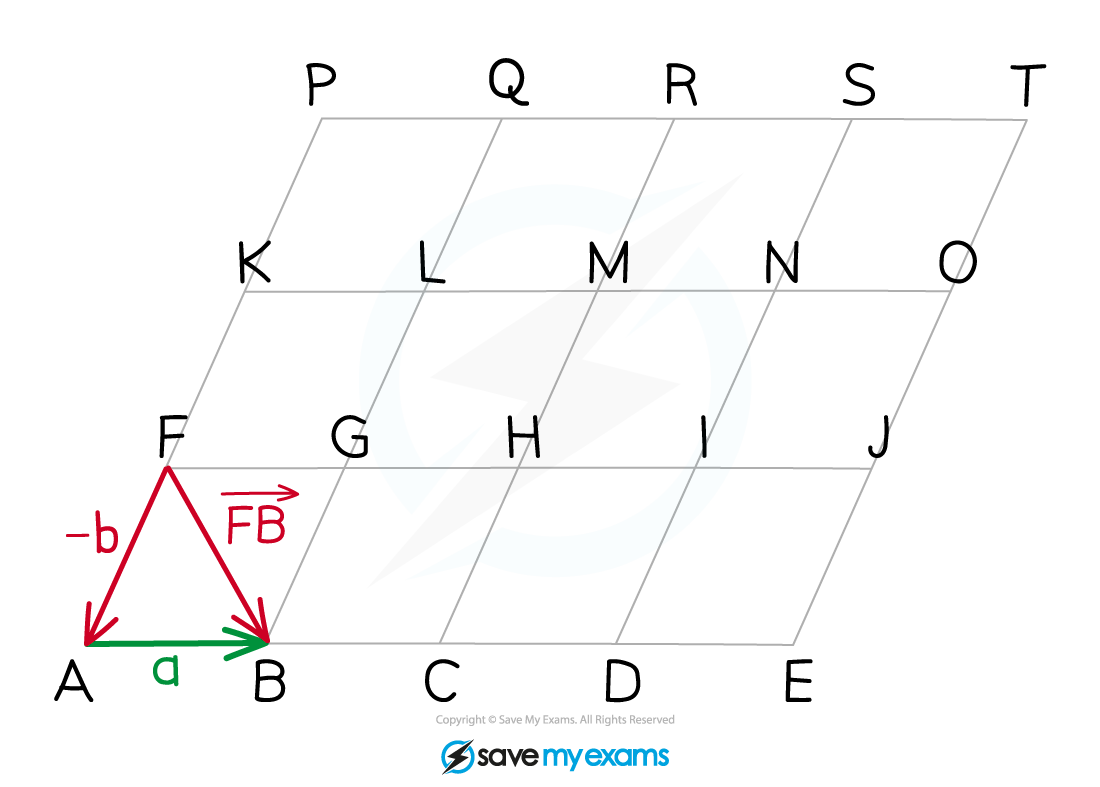• It follows that:• Keeping those things in mind, it is possible to describe any vector that goes from one point to another in the above diagram in terms of a and b

#### Exam Tip

Adding and subtracting vectors follows all the same rules as adding and subtracting letters like x and y in algebra (this includes collecting like terms).It doesn't matter exactly what path you follow through a diagram from starting point to ending point – as long as you add and subtract the general vectors correctly along the path you use, you will get the correct answer.

#### Worked Example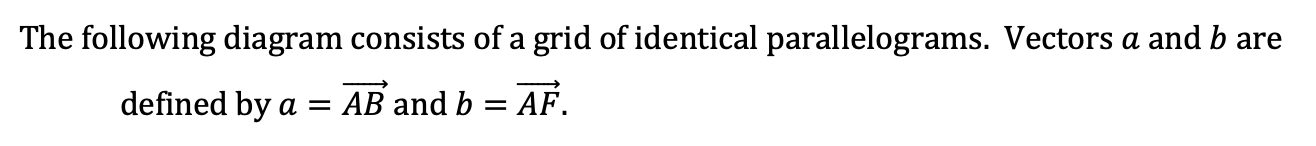#### Worked Example